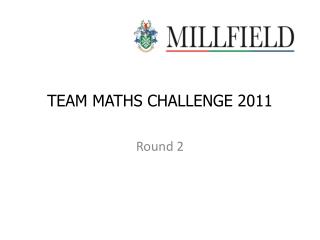DownloadDownload PresentationTEAM MATHS CHALLENGE 2011

# TEAM MATHS CHALLENGE 2011

Télécharger la présentation## TEAM MATHS CHALLENGE 2011

- - - - - - - - - - - - - - - - - - - - - - - - - - - E N D - - - - - - - - - - - - - - - - - - - - - - - - - - -
##### Presentation Transcript

1. TEAM MATHS CHALLENGE 2011 Round 2

2. PRACTICE Multiples of 4 - POP Square numbers - WOOF Fibonacci numbers - FIB [ Each number in the Fibonacci sequence is the sum of the previous two numbers - 1, 1, 2, 3, 5, ...]

3. ACTUAL Triangular number - TING [ A triangular number is the sum of consecutive natural numbers – 1, 3, 6, 10, 15, ...] Multiples of 3 - MEOW Prime digit sum - DIGI [ Example 47: The sum of the digits is prime 4 + 7 = 11]